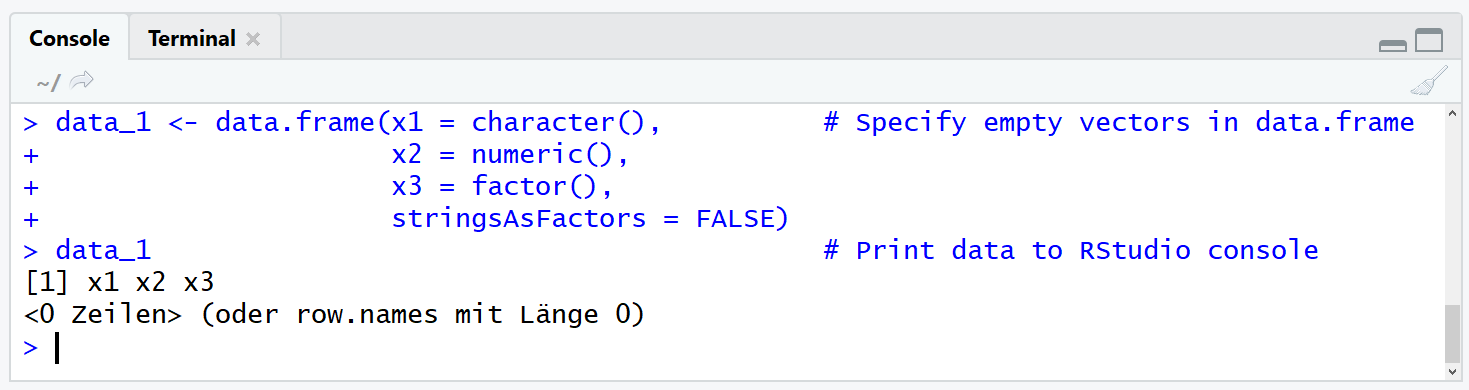# Create Empty Data Frame in R (2 Examples)

In this article, I’ll explain how to create an empty data frame in the R programming language.

I will show you two programming alternatives for the creation of an empty data frame. More precisely, the tutorial will contain the following topics:

Let’s dive in.

## Alternative 1: Initialize Empty Vectors in data.frame Function

In the first example, we will create an empty data frame by specifying empty vectors within the data.frame() function. Consider the following R code:

```data_1 <- data.frame(x1 = character(),          # Specify empty vectors in data.frame
x2 = numeric(),
x3 = factor(),
stringsAsFactors = FALSE)
data_1                                          # Print data to RStudio console```Figure 1: RStudio Console Output is Showing Empty Data Frame.

As you can see based on the RStudio console output, we created an empty data frame containing a character column, a numeric column, and a factor column. Note that we had to specify the argument stringsAsFactors = FALSE in order to retain the character class of our character column. The names of our data frame columns are x1, x2, and x3.

That’s basically it. However, I want to show you another programming alternative in the next example. So keep on reading…

## Alternative 2: Create Matrix with Zero Rows

In the second example, we’ll combine three functions in one line of R code: The matrix function, the data.frame function, and the setNames function. Let’s do this:

```# Create empty data.frame with matrix & setNames functions
data_2 <- setNames(data.frame(matrix(ncol = 3, nrow = 0)), c("x1", "x2", "x3"))```

As you can see based on the previous R syntax, we are nesting the three functions into each other:

• First, we are creating a matrix with zero rows.
• Then, we are converting this matrix to data.frame class.
• And finally, we set the names of our empty data frame with the setNames command.

Note that this R code creates columns with the integer class. You might therefore have to specify the data classes accordingly.

## Video & Further Resources

On my YouTube channel, I have published a video which contains the two programming examples of this tutorial. You can watch it below:

In addition, you might have a look at the other R tutorials of my homepage. You can find some interesting tutorials below:

I hope this tutorial showed how to create a blank and empty data frame in the R programming language. However, let me know in the comments in case you have any further questions. Furthermore, don’t forget to subscribe to my email newsletter to get access to more R tutorials.

Subscribe to the Statistics Globe Newsletter